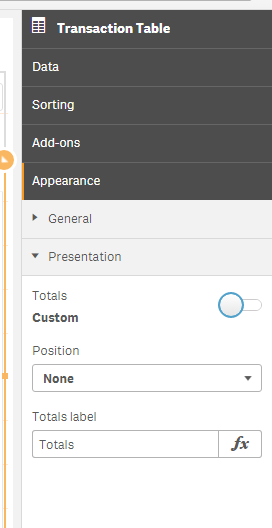# App Development

Announcements
Qlik Cloud Maintenance is scheduled between March 27-30. Visit Qlik Cloud Status page for more details.
cancel
Showing results for
Did you mean:Not applicable

## qlik sense - setting the totals in Pivot Table rows

In QlikView, I know how to set the formula for the totals row in a pivot table as the expression, or sum of rows, etc.

In QlikSense, it defaults to "total", which I assume is the total for the expression. However, in my case, I want it to be sum of rows.

When I click on the "Presentation" icon, I get this:However, when I click on the slider, I get this:The "Position" drop down doesn't drop down, and I can only change the label, not the data.

My rows are Qty, Cost, Extended Cost

my formula are sum(ItemQty), sum(ItemCost), and sum(ItemQty)*Sum(ItemCost). They are fine, except in the

total fields, where the displayed total is clearly incorrect:Clearly, the "Extended Cost" is not equal to the sum of Qty (13563) x the sum of all ItemCosts (\$498k).

I want the sum of rows for the Ext Cost to appear. Is this possible?

3 RepliesPartner - Master III

Is this issue with Pivot table of Straight table.

In Straight Table in Every measure you will get a option as Total Function.. From that drop down select Sum as Function..

In Pivot table YOu will have to write Seperate expression for Total

eg.

if(rowno(),

sum(Aggr(Sum(Qty), Dimension)),

Sum(Qty)

)Contributor II

Thank you for being precise and providing the details on where the follow set expression can be used. This was very helpful to me :). Thanks again!.MVP

Looks like you've already gotten a working response, but I'm curious: why not write the expression as Sum(QTY*ItemCost) in the first place?

As for the more complex option, another alternative should be:

Sum(Aggr(Sum(QTY)*Sum(ItemCost),Dimensions))Tags
Community Browser